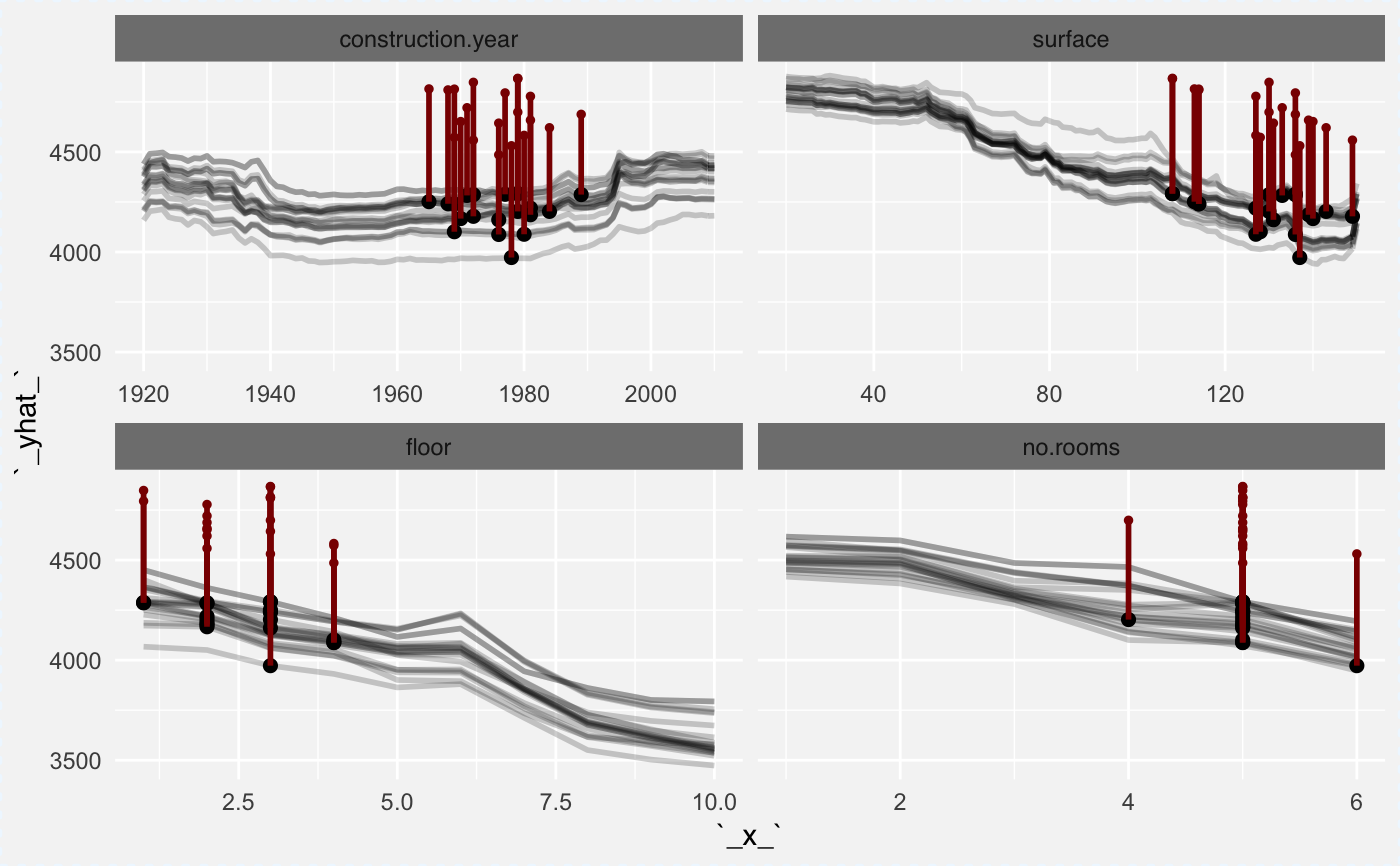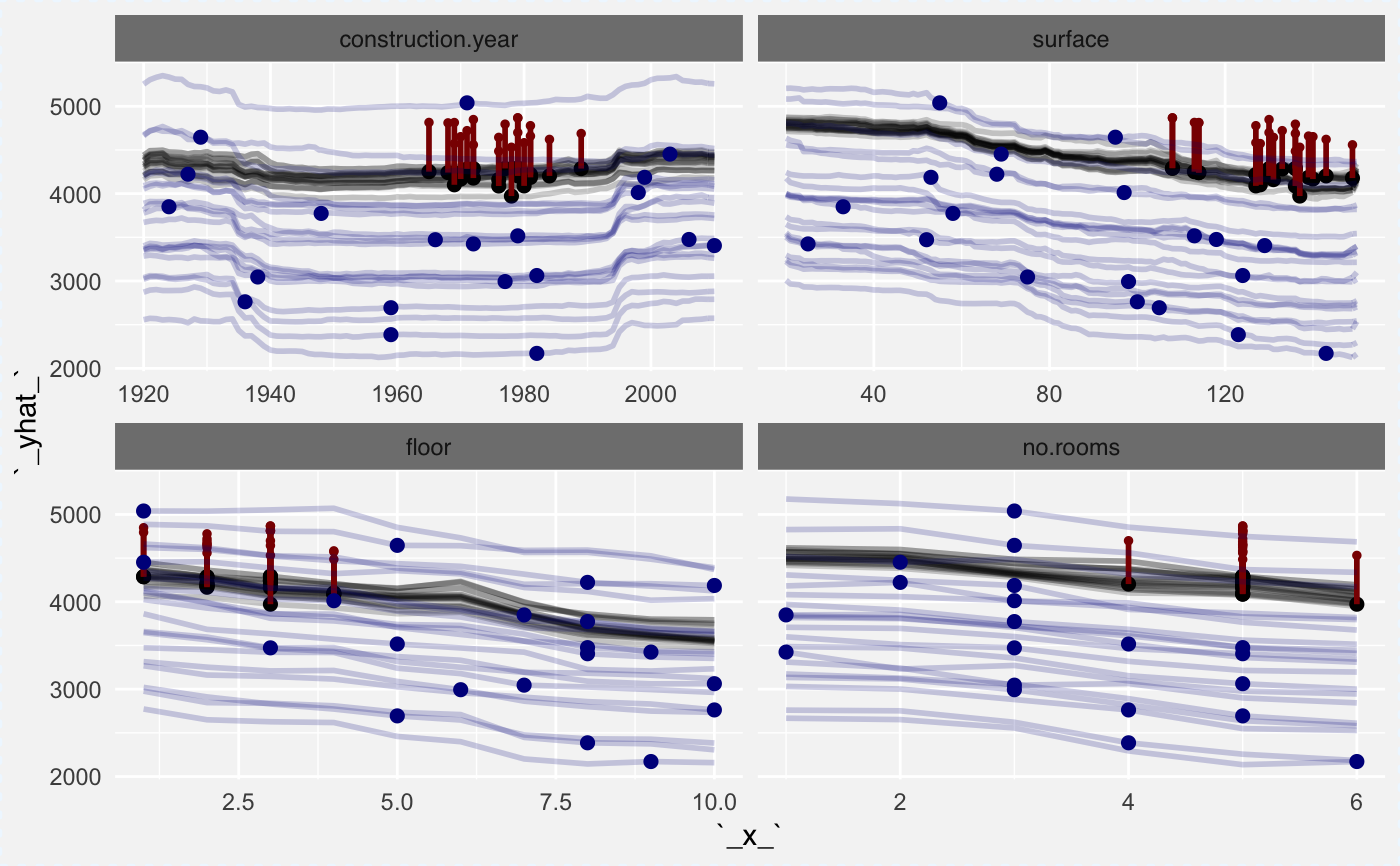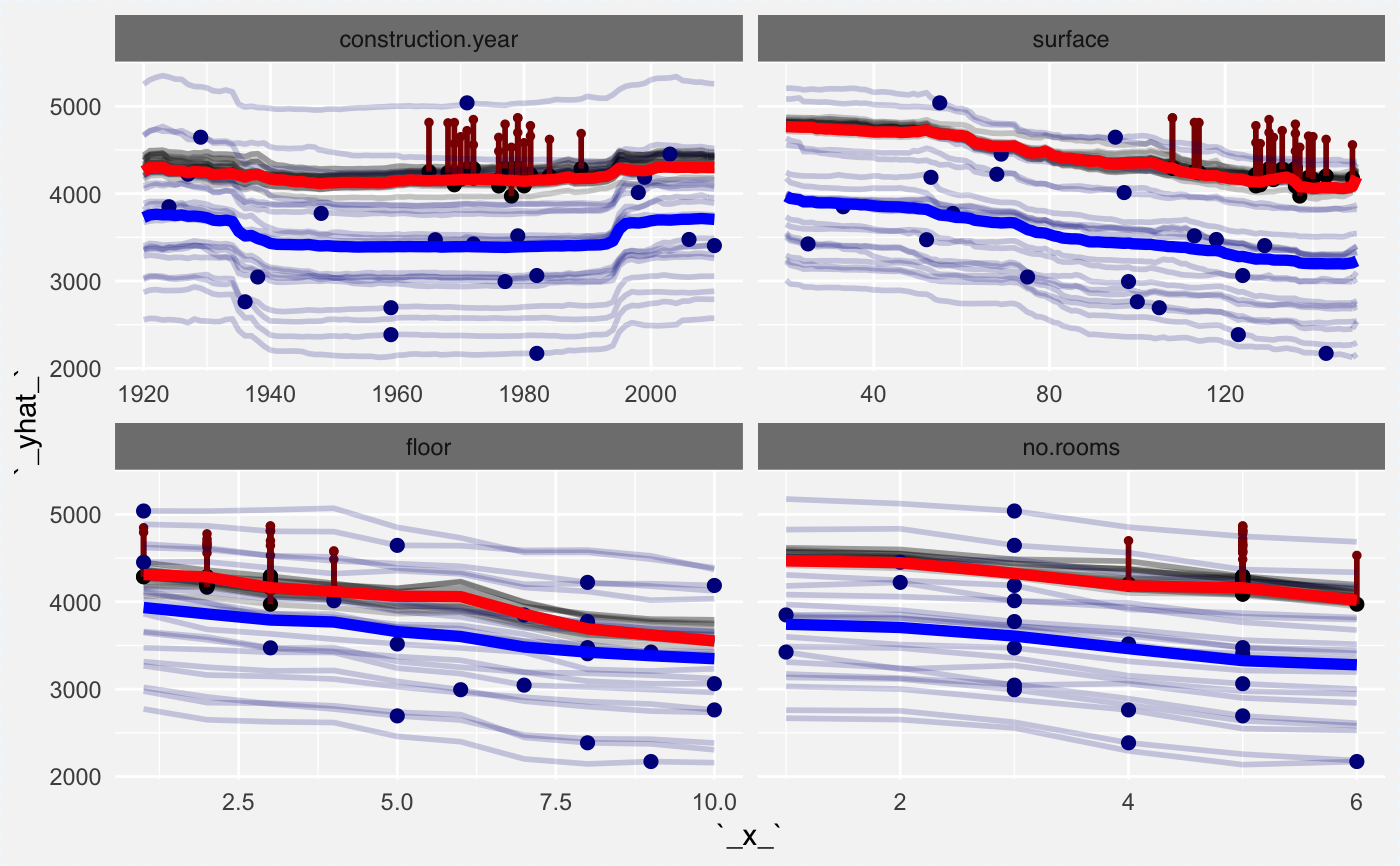Function ceteris_paribus_layer() adds a layer to a plot created with plot.ceteris_paribus_explainer() plots. Various parameters help to decide what should be plotted, profiles, aggregated profiles, points or rugs.

ceteris_paribus_layer(x, ..., size = 1, alpha = 0.3, color = "black",
size_points = 2, alpha_points = 1, color_points = color,
size_rugs = 0.5, alpha_rugs = 1, color_rugs = color,
size_residuals = 1, alpha_residuals = 1, color_residuals = color,
only_numerical = TRUE, show_profiles = TRUE,
show_observations = TRUE, show_rugs = FALSE,
show_residuals = FALSE, aggregate_profiles = NULL, as.gg = FALSE,
facet_ncol = NULL, selected_variables = NULL, init_plot = FALSE)

## Arguments

x a ceteris paribus explainer produced with function ceteris_paribus() other explainers that shall be plotted together a numeric. Size of lines to be plotted a numeric between 0 and 1. Opacity of lines a character. Either name of a color or name of a variable that should be used for coloring a numeric. Size of points to be plotted a numeric between 0 and 1. Opacity of points a character. Either name of a color or name of a variable that should be used for coloring a numeric. Size of rugs to be plotted a numeric between 0 and 1. Opacity of rugs a character. Either name of a color or name of a variable that should be used for coloring a numeric. Size of line and points to be plotted for residuals a numeric between 0 and 1. Opacity of points and lines for residuals a character. Either name of a color or name of a variable that should be used for coloring for residuals a logical. If TRUE then only numerical variables will be plotted. If FALSE then only categorical variables will be plotted. a logical. If TRUE then profiles will be plotted. Either individual or aggregate (see aggregate_profiles) a logical. If TRUE then individual observations will be marked as points a logical. If TRUE then individual observations will be marked as rugs a logical. If TRUE then residuals will be plotted as a line ended with a point function. If NULL (default) then individual profiles will be plotted. If a function (e.g. mean or median) then profiles will be aggregated and only the aggregate profile will be plotted if TRUE then returning plot will have gg class number of columns for the facet_wrap(). if not NULL then only selected_variables will be presented technical parameter, do not use.

a ggplot2 object

## Examples

library("DALEX")library("randomForest")
set.seed(59)

apartments_rf_model <- randomForest(m2.price ~ construction.year + surface + floor +
no.rooms + district, data = apartments)

explainer_rf <- explain(apartments_rf_model,
data = apartmentsTest[,2:6], y = apartmentsTest$m2.price) apartments_small_1 <- apartmentsTest[1,] apartments_small_2 <- select_sample(apartmentsTest, n = 20) apartments_small_3 <- select_neighbours(apartmentsTest, apartments_small_1, n = 20) cp_rf_y1 <- ceteris_paribus(explainer_rf, apartments_small_1, y = apartments_small_1$m2.price)
cp_rf_y2 <- ceteris_paribus(explainer_rf, apartments_small_2, y = apartments_small_2$m2.price) cp_rf_y3 <- ceteris_paribus(explainer_rf, apartments_small_3, y = apartments_small_3$m2.price)

tmp <- plot(cp_rf_y3, show_profiles = TRUE, show_observations = TRUE,
show_residuals = TRUE, color = "black",
alpha = 0.2, color_residuals = "darkred",
selected_variables = c("construction.year", "surface"))

tmp <- plot(cp_rf_y3, show_profiles = TRUE, show_observations = TRUE,
show_residuals = TRUE, color = "black",
alpha = 0.2, color_residuals = "darkred")

tmptmp +
ceteris_paribus_layer(cp_rf_y2, show_profiles = TRUE, show_observations = TRUE,
alpha = 0.2, color = "darkblue")tmp +
ceteris_paribus_layer(cp_rf_y2, show_profiles = TRUE, show_observations = TRUE,
alpha = 0.2, color = "darkblue") +
ceteris_paribus_layer(cp_rf_y2, show_profiles = TRUE, show_observations = FALSE,
alpha = 1, size = 2, color = "blue", aggregate_profiles = mean) +
ceteris_paribus_layer(cp_rf_y1, show_profiles = TRUE, show_observations = FALSE,
alpha = 1, size = 2, color = "red", aggregate_profiles = mean)